International Journal of Discrete Mathematics
Volume 1, Issue 1, December 2016, Pages: 30-32

Algorithm of Search of Large Prime Numbers

Kochkarev Bagram Sibgatullovich

Department of Mathematics and Mathematical Modeling, Institute of Mathematics and Mechanics Named After N. I. Lobachevsky, Kazan (Volga Region) Federal University, Kazan, Russia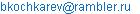Kochkarev Bagram Sibgatullovich. Algorithm of Search of Large Prime Numbers. International Journal of Discrete Mathematics. Vol. 1, No. 1, 2016, pp. 30-32. doi: 10.11648/j.dmath.20160101.15

Received: December 3, 2016; Accepted: December 22, 2016; Published: January 17, 2017

Abstract: The large number of problems in the theory of the numbers possessing one characteristic sign called by us binary mathematical statements from the natural parameter which in the time of Pythagoras and Euclid still aren’t solved was prompted to us that the reason of such situation should be looked for in the mathematics bases. We have entered concept of the binary mathematical statement depending from natural parameter and have specified axiomatic of natural numbers of Peano, having added one axiom called by us the axiom of descent which is interpretation of a so-called method of descent of Fermat by means of which he has proved the Great Hypothesis for a special case of n=4. By means of a descent axiom we managed to receive a large number of the results published in Russian. Wishing to expand a readership, we have decided to give the review of our results which are already published in Russian without proofs and to add new results among which the algorithm of search of large prime numbers is dominating with proofs.

Keywords: Binary Mathematical Problem, Axiom of Descent, Algebraic Equation, Diophantine Equation

Contents

1. Introduction

The present article represents the review of the results received by the author in work [1-5], devoted to the subject touched by Pierre Fermat in his Great Hypothesis and remarks given on the fields of the book "Arithmetician" of Diophantus and published [6, 72] in 1670 by the son Fermat Clement-Samyuel in the book under the name "The Diophantine Arithmetics Contening Notes P. of De Fermat". Except the results published in [1-5] article contains also some not published results of the author yet.

2. Method

Definition 1. . The mathematical statement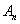, depending from natural parameter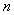we will call binary if for any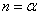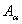value has one or the other value: truth or lie.

In case of binary statementsFermat has invent [7, 70] a so-called method of descent by means of which he has proved that a class of the Diophantine equationsfor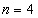has no decision in a ring of integers. Method of Fermat’s descent it is expedient to formulate in the form of an axiom of descent : let- the binary mathematical statement depending from natural parameterit that 1) there is an algorithm which for any valuegive the answer to the question " the statement ofis true or false?", 2) for some final series of values of the parameter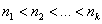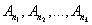are true and for any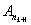according to the assumption is false. Then the statement ofis true for an infinite set of values.

(1). In  about infinity of a number of twins and Hardi and Littlewood [8,367] problem of the proof of existence of an infinite set of the three of prime numbers is easily proved:.

(2). Definition 2. (Pythagoras) The natural numberis called perfect if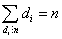, where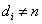is divider of number.

Theorem 1 . All odd natural numbers are not perfect.

Theorem 2. A natural number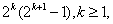is perfect if and only if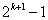is a prime number.

It is known [8, 37] that prime numbers of a type of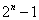in literature are called Mersenn’s numbers, the contemporary and correspondent of P. Fermat [7, 69].

In  by means of a descent axiom we have proved the theorem.

Theorem 3 . The set of numbers of Mersenn is infinite.

Corollary 1 . The set of perfect numbers is infinite.

Corollary 2 . The sequence of numbers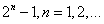contains an infinite set of prime numbers.

By means of a descent axiom we have also easily proved (any prime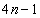number never is the sum of two squares) statement for which proof required to Euler [6, 73] seven years of work.

(3). Theorem 4 . (Binary problem of Goldbach-Euler). Each even number, since 4, can be presented in the form of the sum of two prime numbers

Corollary 3 . (Euler) Each odd number, since 7, can be presented in the form of the sum of three prime numbers.

Corollary 4 . Whatever even number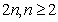, was, there will be a prime number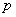such that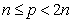and ifis compound, then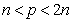and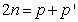, where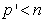is a prime number.

Theorem 5 . Slightly excess numbers [6,29] don’t exist.

3. New Results

Corollary 5 to theorem 2. Mersenn’s numbers are never representable in the form of the sum of two squares.

Proof. Letbe Mersenn’ number, i.e. it is a prime number. Obviously,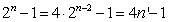.

In Fermat’s notes there is a statement [9, 11], that all numbers of a type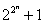are primes but Fermat is the statement has accompanied with a mark that he has no him the satisfactory proof. With some specification of this statement easily by means of an axiom of descent the theorem is proved.

Theorem 6. The sequence of numbers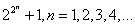contains infinitely many prime numbers.

Proof. It is easy to be convinced thatgives prime numbers for natural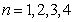and for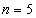Euler has shown [9, 11], that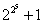is a composite number. We will assume the theorem for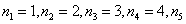is fair, and for any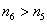is a composite number. Then on an axiom of descent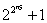is also a composite number that contradict the inductive assumption. The received contradiction proves the theorem. We will notice that according to [8, 38].

Definition 3. We will call prime numbers of a type,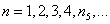Fermat’s numbers.

We will consider the sequence of numbers. Obviously, this sequence of numbers includes the sequence of numbers of a type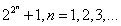, and consequently Fermat’s numbers in the structure. It is easy to show [8, 35] that the prime numbers entering these sequences coincide and as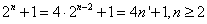they according to the theorem 2  are representable in the form of the sum of two squares. Ifhas an odd divider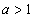, then from [8, 35] follows that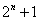is a composite number. From here also follows.

Theorem 7. Ifis a prime number,, thenis the work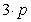, whereis a prime number.

Proof. We will assume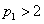there is the first prime number in a natural number sequence. In this case we have. If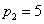, then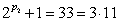. We will assume for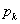takes place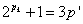, where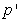is a prime number, and for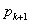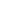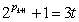, where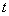is a composite number. Then on an axiom of descent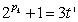, whereis also a composite number, and it contradicts the inductive assumption. The received contradiction proves the theorem.

The proved theorem to us delivers an algorithm of search of large prime numbers. Ifis a prime number,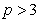, then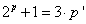, where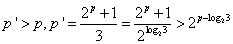.

4. Class of Diophantine Equations of Fermat

It is known [7, 70] that the Diophantine equation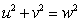which solution Fermat has studied from the book "Arithmetician" of Diophantus has formed for him a basis for the formulation of the well-known hypothesis. In  we have offered a method of the solution of the equationof Pythagoras by the reduction him to a class of the algebraic equations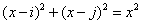from two natural parameters.

Fermat has generalized the specified equation and on fields of the book "Arithmetician" of Diophantus [7, 70] against of equationhe has written: "it is impossible to spread out neither a cube to two cubes, nor a biquadrate on two biquadrates, and in general any degree, big a square on two degrees with the same indicator. I have opened to it really wonderful proof, but this field for it is very narrow".

In , we have proved stronger statement, than the statement formulated by P. Fermat.

Theorem 8 . The Diophantine equation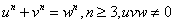has no decision not only in a ring of integers, but also in the field of rational numbers.

We will notice that the proof of the theorem 8 at the same time give an algorithm of the proof of this statement for any concretewhereas others, starting with Euler proved this statement only for some.

In 1995 article has been published in Annals of Mathematics (USA)  which has caused a lot of noise in the press and some publications, for example, in the form of the monograph  and on the Internet .

From theorem 8 follows that this article, unfortunately, wrong as the elliptic curve of Frey according to the theorem 8 in the nature doesn’t exist.

In the history of mathematics such cases happened: so, for example, the 21 st problem of D. Gilbert "has been positively solved" at the beginning of the 20 th century by the mathematician E. Plemel, but in 70 years the mathematician A. A. Bolibrukh has noticed an error in E. Plemel’s proof and has solved D. Gilbert’s problem has the negative decision .

We weren’t satisfied with the proof of the specified statement (the theorem 8) and have closed a question of solution of the Diophantine equation of Fermat.

Theorem 9 . The Diophantine equation of Fermat for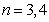has decisions in radicals.

Theorem 10 . The Diophantine equation of Fermat for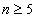algorithmically unsoluble.

Obviously, justice of the Great Hypothesis of Fermat as the result follows from the results received above.

At last, in  we have formulated a problem: to find natural numbers pressing between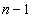anddegrees.

This problem has the infinite number of decisions for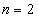, the unique decision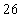for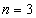(Fermat) and there are no decisions for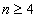. Though this problem has been published by us in 2014  and we have thrown a call to fans and to mathematical community to prove it, we still haven’t received a response to our call.

5. Conclusion

From the history of mathematics is well known  that many mathematical problems, seemingly insurmountable, with proper revision foundations of mathematics are solvable. Analisis of this type of problems in the theory of numbers helped us by introducing the concept of binary mathematical statements and corresponding adjustements of axioms of natural numbers Peano, solve the problems, which the age of some of them reaches more than 2500 years.

References

1. Kochkarev B. S. Ob odnom klasse algebraicheskikh uravnenii, ne imejutchikh ratsionalnykh reshenii. Problems of modern science and education. 2014. 4 (22), s. 9-11 (in Russian).
2. Kochkarev B. S. Ob odnom svoistve naturalnykh chisel. Problems of modern science and education. 2014. 7 (25), s. 6-7 (in Russian).
3. Kochkarev B. S. Svedenie odnogo Diophantova uravnenija k klassu algebraicheskikh uravnenii ot dvukh naturalnykh parametrov. Problems of modern science and education. 2015. 7 (37), s. 6-7 (in Russian).
4. Kochkarev B. S. K metodu spuska Ferma. Problems of modern science and education. 2015. 11 (41), s. 7-10 (in Russian).
5. Kochkarev B. S. Problema bliznetsov i drugie binarnye problem. Problems of modern science and education 2015. 11 (41), s. 10-12 (in Russian).
6. Singkh S. Velikaya teorema Ferma. MTSNMO. 2000. s. 288.
7. Samin D. K. Sto velikikh uchenykh. Moskow, "Veche". 2001. S. 592 (in Russian).
8. Bukhshtab A. A. Teoriya chisel. Moskow. Izd. "Prosvetchenie". 1966, s. 384 (in Russian).
9. Postnikov M. M. Vvedenie v teoriju algebraicheskikh chisel. Moskow, "Nauka" Glavnaya redaktsiya fiziko- matematicheskoy literatury, 1982. s. 240 (in Russian).
10. Kochkarev B. S. About one binary problem in a class of algebraic equations and her communication with the Great Hypothesis of Fermat. International Journal of Current Multidisciplinary Studies. Vol. 2, Issue, 10, pp. 457-459, October, 2016.
11. Wiles A. Modular elliptic curves and Fermat’s Last Theorem. Annals of Mathematics, v. 141 Second series 3 May 1995 pp.445-551.
12. Abrarov D. Teorema Ferma: fenomen dokazatelstva Uailsa. http: //polit.ru/article/2006/12/28/abrarov/.
13. Kudryavtsev L. D. O matematike//Tezisy dokladov Mejdunarodnoi nauchno-obrazovatelnoi konferentsii 23-27 marta 2009 goda. Nauka v Vuzakh: matematika, fizika, informatika. Moskow, RUDN, 2008 (in Russian).
14. Kochkarev B. S. Otlichitelnoe svoistvo naturalnykh chisel v razlichnykh geometriyakh. Problems of modern science and education 2015. 5 (35), s. 6-9 (in Russian).
15. Laptev B. L. Nikolai Ivanovich Lobachevsky. Izd. Kazanskogo universiteta. 1976. s. 136 (in Russian).

 Contents 1. 2. 3. 4. 5.
Article ToolsAbstractPDF(147K)